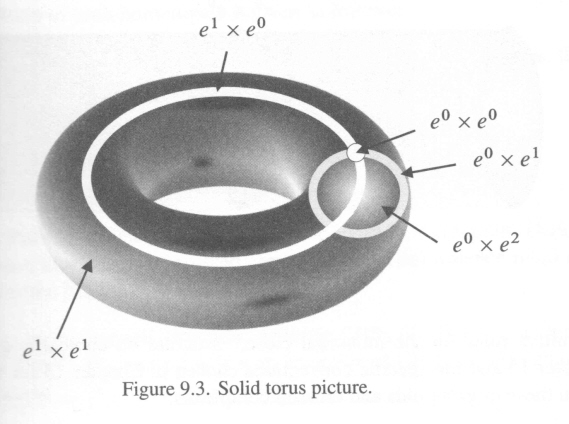Visualizing products of $CW$ complexes

I'm learning about products of CW complexes. The sources I've seen talk about the matter as follows: given topological spaces $X$ and $Y$ with a given CW decomposition, we can then form a CW decomposition of $X \times Y$ by understanding the characteristic maps as the product maps.

OK, but starting with $X \times Y$ and then breaking it down into cells assumes (perhaps) that we have some notion of what $X \times Y$ looks like. I'm interested in understanding the structure of $X \times Y$ by inductively building a cell complex. For the most part, the attaching maps seem pretty hard to wrap my head around. OK, these things are hard to visualize, but it's nice to try.

Suppose I don't know what $S^1 \times S^2$ is like (I don't). I try to get a handle on its cell decomposition, using for $S^1$ and $S^2$ the decompositions with two cells each. To build it, I'd start with a $0$ cell, attach a $1$ cell like a circle, and then attach the two skeleton which is a single $D^2$ with boundary collapsed to a point. So the two-skeleton is $S^1 \vee S^2$.

Now I should attach a solid tube like a cannoli, and I'm trying to understand the attaching map. The boundary of the tube has two flat sections $D^2$ and one curvy section $S^1 \times I$. I need to attach the flat parts to the sphere and the curvy part to the circle. Essentially I have a solid doughnut where I identify every boundary point that has the same position in the big circle?

Is it productive for me to try to visualize things this way? I'm kind of banging my head against it.

• I find it difficult to visualize interesting spaces from their CW-decomposition; if I really want to visualize the CW decomposition, I tend to work backwards, and start by visualizing the space instead, emedding the skeleta inside it. In particular, if I didn't know what a torus looked like, I would have trouble building a picture from the CW decomposition alone. – user98602 May 1 '15 at 23:13
• I like to think of $S^2\times S^1$ as follows. First think of $S^2\times I$. This is a thickened sphere. To turn $I$ into $S^1$, you need to identify its two endpoints. So $S^2\times S^1$ is a thickened sphere with inside and outside glued together. If you imagine walking around in the thickened sphere once you hit the outer boundary you reappear at the inner boundary. – Cheerful Parsnip May 18 '17 at 15:13

In most cases visualizing CW-complex skeleta-by-skeleta in the way you described is pointless (look at Mike's comment). However there is a formula for doing that, namely:

let's denote by $\chi_{X^{(n)}}^{i} : S^{n-1} \to X^{n-1}, \chi_{Y^{(n)}}^{j} : S^{n-1} \to Y^{n-1}$ the attaching maps where $i \in I_{n}, j \in J_{n}$ are indices of them. We are defining:

$$(X \times Y)^{(n)} = \bigcup_{k + l = n} X^{(k)} \times Y^{(l)}$$

with attaching maps: $$\chi_{X \times Y ^{(n)}} = \bigsqcup_{k + l = n - 1} \bigsqcup_{i \in I_{k}, j \in J_{l}} \chi_{X^{(n)}}^{i} \times \chi_{Y^{(n)}}^{j}$$

observe that we are attaching all the $n$-cells here at once and that these products of attaching maps can be defined in canonical way because of the following observation:

$$(D^{k + l}, S^{k + l - 1}) = (D^{k} \times D^{l}, S^{k - 1} \times D^{l} \cup D^{k} \times S^{l - 1}).$$

Try to decompose $S^1 \times S^2$ in that way. I doubt whether there is a better method for insight into product skeleta.

• There are some things that confuse me about your answer. First of all, I think $I_j$ is indexing the $j$ cells, but then in the attaching maps, you write $\chi_{X^{(n)}}^i \times \chi_{Y^{(n)}}^j$ where $i \in I_k$ and $j \in J_l$, but it would seem we want $\chi_{X^{(k)}}^i \times \chi_{Y^{(l)}}^j$. Second, what does the notation $(D^{k+l}, S^{k+l-1})$ mean? Third, you write ($X \times Y)^{(n)} = \cup X^{(k)} \times Y^{(l)}$, not disjoint union, but how are we supposed to get a handle on the points shared by these sets if we don't have a picture of $X \times Y$ yet? – Eric Auld May 2 '15 at 21:22

I think it is helpful to have a picture. Here is one of a solid torus with cell decomposition:taken from the book Nonabelian Algebraic Topology.

The algebraic version of a product cell decomposition is one of the harder parts of that book, involving a complicated tensor product. One reason for the complications is that the product $E^m \times E^n$, where $E^m$ has cell decomposition $e^0 \cup e^m$, is not so simple, as Adam's answer shows. The book uses also a family of cubes $I^m$, which are somewhat more convenient for products since $I^m \times I^n\cong I^{m+n}$. However to get an algebraic form of the relations between these two, i.e. the $E^m$ and the $I^m$, is one of the harder parts of the book; it should be said that this relation geometrically goes way back in homotopy theory.

Somewhat related is the description of a $3$-sphere as the subspace of $\mathbb R^4$ given by $x_1^2 + x_2^2 + x_3^2 +x_4^2=1$ as the union of two solid tori one of which is given by $x_1^2 +x_2^2 \leqslant 1/2$.

To lower the tone a bit, but which might help your visualisation, there is an amusing trick with the bottom part of a striped pyjama. Carefully sew the bottom two legs together along the bottom edges. Through the top hole, you can turn the sewn pyjama inside out. What happens? Does anyone know of a web link on this?